Estimation (approximate) of the size of contracts in Ethereum

As some of you may know, contracts in Ethereum have storage. Contracts write into their storage by using opcode SSTORE, and read it back using opcode SLOAD. Contract storage is modelled as a mapping, i.e. key => value. Both keys and values are 256-bit (32 bytes) values. For each contract, Ethereum tracks so called storage root, 32-byte string, which is the root of the Merkle Patricia tree, composed of those storage items “key => value”. In order to make that Markle Patricia tree more balanced, it is actually composed of items “keccak256(key) => value” and not simply “key => value”. Here, function keccak256 is used for randomisation, under the “random oracle” assumption.

Unfortunately, Ethereum does not track the size of the contract storage for each contract. This would be useful for at least two things:

1. Advanced snapshot sync protocols (need for such protocols described here)
2. Storage rent prepayment as a part of State Fees (rent)

Here I will mostly be taking about (2). In the latest published State Fees proposal, there is a mechanism for charging contract storage rent prepayments, that relies on the knowledge of the contract storage size: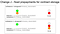Page 37 of the State Fees proposal (version 3)

Depending on the relation between rentbalance and storagesize, storage prepayment is either charged or not in the case of SSTORE modifying an existing storage item (orange circle). This measure is designed to preclude hoarding of the contract storage ahead of the hard fork that makes SSTORE more expensive.

On the other hand, change J is believed to be a pre-requisite for the safe lifting of the block gas limit: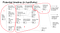Page 8 of the State Fees proposal (version 3)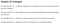Page 9 of the State Fees proposal (version 3)

The fact that Ethereum does not currently track the storage sizes of contract, means that the storage rent prepayments (and safe block gas limit increase) were slated for the second hard fork (safe introduction of the accurate contract storage sizes requires two hard forks).

However, if there was a way to estimate the contract sizes approximately, and if we agreed that these were still useful to decide on the prepayments, we could bring the change J forward into the 1st hard fork: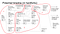Modified timeline that brings block gas limit increase closer?

Now, how could this estimation work?

Since keccak256 is assumed to be randomising, for n storage keys key_1, key_2, …, key_n, the distribution of keccak256(key_1), keccak256(key_2), …, keccak256(key_n) would be quite uniform in the range of numbers 0..2²⁵⁶-1. Therefore, on average, the distance between two subsequent key hashes, would be 2²⁵⁶/n. We can reverse the problem, and try to estimate the average distance between two subsequent key hashes, let’s say, avg_diff, and then estimate n as 2²⁵⁶/avg_diff. One can show this more rigorously, based on modelling the sequence of key hashes as a Poisson process, then realising that the difference between two events would follow an exponential distribution, and, finally, deriving the maximum likelihood estimator for n, which basically tells us to estimate n as 2²⁵⁶/avg_diff.

In order to see how accurate such estimates would be, I chose one particular method for estimating avg_diff. Most probably, another, simpler and better method can be found, and I hope for someone to help me do it.

We represent the range 0..2²⁵⁶-1 as a circle (we wrap the range so that 0 equals 2²⁵⁶), and generate certain number of equidistant numbers, called “probes”. In the illustration above, there are 5 probes, shown as green rays. The first probe is chosen using some pseudo-random number. For example, we can take the storage root for that purpose. For each probe, we look for the first keyhash (blue ray) equal or after the probe, and then a certain number of subsequent keyhashes (also blue rays) after that (in the illustration, this number is 2). It is likely that in the implementations of Ethereum, finding the keyhash next to the probe is more expensive operation than finding the next keyhash after a known one.

As a result of this “probing”, we have number of samples. In this illustration above, there are 5x2 = 10 samples. Out of these samples, we calculate avg_diff as a simple average.

Experimental results

I have taken the state of Ethereum main net at block 7293802 (March 3rd 2019), and analysed all contracts and their storage. As suggested before, the storage root was used as a seed to choose the first probe. The number of probes varied from 1 to 19, and number of items explored after each probe varied from 1 to 49 (there were 931 estimations done for each contract). For each estimation, the relative error was computed in percentages of the actual storage size. Maximum of the relative errors over all the contracts were gathered in this heatmap table (rows are number of probes, columns are number of items explored after each probe):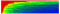Relative estimation errors for all contracts

In order to make better sense of this result, similar heatmap tables were calculated for groups of contracts depending on their storage size: